# 知识点小结

• 重载的概念是：方法名称相同，参数个数、次序、类型不同因此重载对返回值没有要求，可以相同，也可以不同，但是如果参数的个数、类型、次序都相同，方法名也相同，仅返回值不同，则无法构成重载如：
``````//这2个方法不能构成重载，会有编译错误。
public int A(int i);
public double A(int i);

//这2个方法可以形成重载
public int A(int i);
public double A(double i);``````

• 如果我们有两个方法如下，当我们调用：test(1) 时，编译器无法确认要调用的是哪个。方法的返回值只是作为方法运行之后的一个“状态”，但是并不是所有调用都关注返回值，所以不能将返回值作为重载的唯一区分条件。
``````// 方法1
int test(int a);
// 方法2
long test(int a);``````

## 抽象类（abstract class）和接口（interface）的区别

• 抽象类只能单继承，接口可以多实现。
• 抽象类可以有构造方法，接口中不能有构造方法。
• 抽象类中可以有成员变量，接口中没有成员变量，只能有常量（默认就是 public static final）。
• 抽象类中可以包含非抽象的方法，在 Java 7 之前接口中的所有方法都是抽象的，在 Java 8 之后，接口支持非抽象方法：default 方法、静态方法等。Java 9 支持私有方法、私有静态方法。
• 抽象类中的方法类型可以是任意修饰符，Java 8 之前接口中的方法只能是 public 类型，Java 9 支持 private 类型。

## wait() 和 sleep() 方法的区别

1. 来源不同：sleep() 来自 Thread 类，wait() 来自 Object 类。
2. 对于同步锁的影响不同：sleep() 不会该表同步锁的行为，如果当前线程持有同步锁，那么 sleep 是不会让线程释放同步锁的。wait() 会释放同步锁，让其他线程进入 synchronized 代码块执行。
3. 使用范围不同：sleep() 可以在任何地方使用。wait() 只能在同步控制方法或者同步控制块里面使用，否则会抛 IllegalMonitorStateException。
4. 恢复方式不同：两者会暂停当前线程，但是在恢复上不太一样。sleep() 在时间到了之后会重新恢复；wait() 则需要其他线程调用同一对象的 notify()、nofityAll() 才能重新恢复。

## 为什么要使用线程池？为什么不直接new个线程？

1. 降低资源消耗。通过重复利用已创建的线程，降低线程创建和销毁造成的消耗。
2. 提高响应速度。当任务到达时，任务可以不需要等到线程创建就能立即执行。
3. 增加线程的可管理型。线程是稀缺资源，使用线程池可以进行统一分配，调优和监控。

## List、Set、Map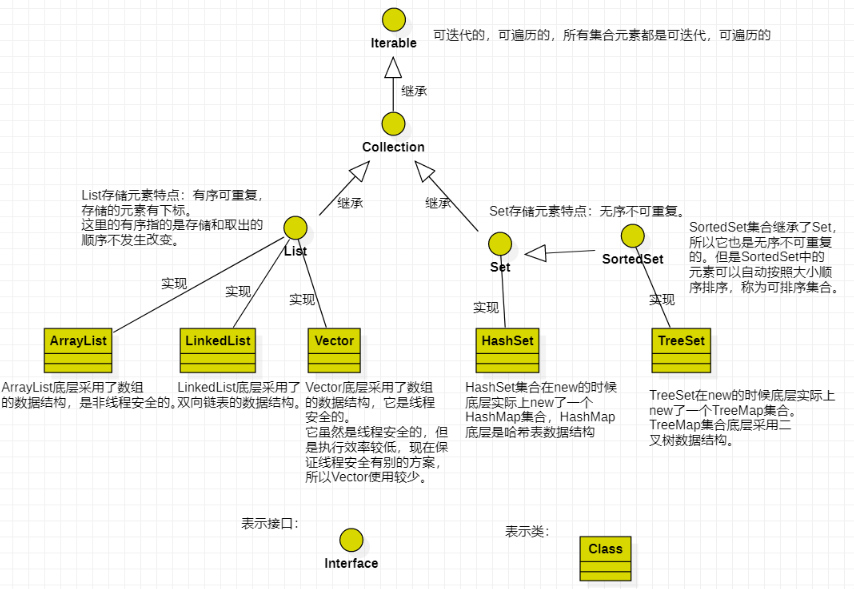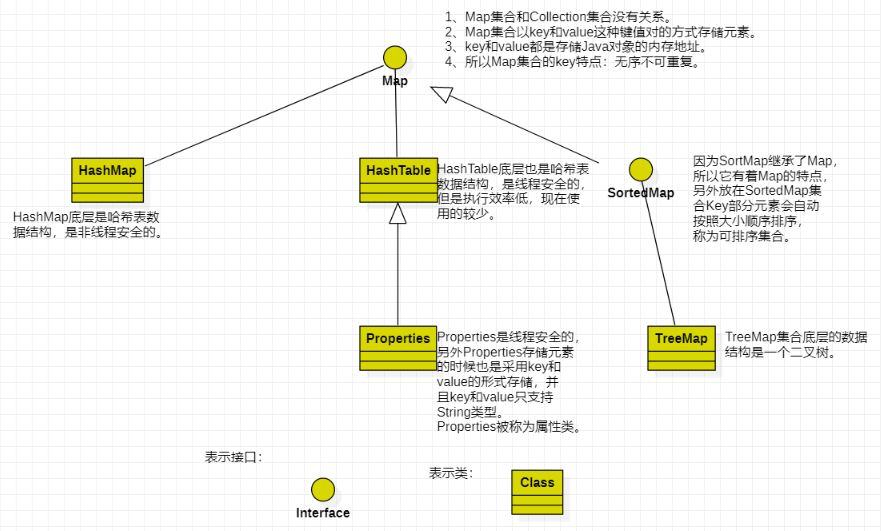## HashMap 和Hashtable 的区别

1. HashMap 允许 key 和 value 为 null，Hashtable 不允许。
2. HashMap 的默认初始容量为 16，Hashtable 为 11。
3. HashMap 的扩容为原来的 2 倍，Hashtable 的扩容为原来的 2 倍加 1。
4. HashMap 是非线程安全的，Hashtable是线程安全的。
5. HashMap 的 hash 值重新计算过，Hashtable 直接使用 hashCode。
6. HashMap 去掉了 Hashtable 中的 contains 方法。
7. HashMap 继承自 AbstractMap 类，Hashtable 继承自 Dictionary 类。

## 类加载的过程

1. 类加载的过程包括：加载、验证、准备、解析、初始化，其中验证、准备、解析统称为连接。
2. 加载：通过一个类的全限定名来获取定义此类的二进制字节流，在内存中生成一个代表这个类的java.lang.Class对象。
3. 验证：确保Class文件的字节流中包含的信息符合当前虚拟机的要求，并且不会危害虚拟机自身的安全。
4. 准备：为静态变量分配内存并设置静态变量初始值，这里所说的初始值“通常情况”下是数据类型的零值。
5. 解析：将常量池内的符号引用替换为直接引用。
6. 初始化：到了初始化阶段，才真正开始执行类中定义的 Java 初始化程序代码。主要是静态变量赋值动作和静态语句块（static{}）中的语句。

## 饿汉模式、懒汉模式和静态内部类（延迟初始化占位类）

``````//线程安全、非懒加载、效率高。
public class Singleton {

// 1.饿汉
private static Singleton instance = new Singleton();

private Singleton() {}

public static Singleton getInstance() {
return instance;
}
}``````

``````//线程安全、懒加载、效率低。
public class Singleton {

// 2.懒汉
private static Singleton instance;

private Singleton() {}

public static synchronized Singleton getInstance() {
if (instance == null) {
instance = new Singleton();
}
return instance;
}
}``````

``````//线程安全、懒加载、效率高。
public class Singleton {

// 4.静态内部类
private static class Holder {
private static final Singleton INSTANCE = new Singleton();
}

private Singleton() {}

public static final Singleton getInstance() {
return Holder.INSTANCE;
}
}``````

## 排序算法

• 冒泡排序: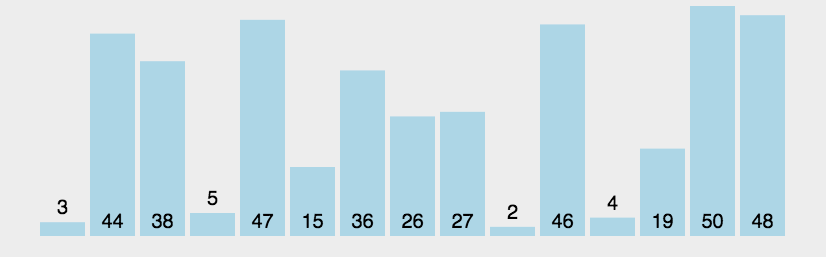``````public static void bubbleSort(int[] array) {
if (array == null || array.length == 0) {
return;
}
for (int i = 0; i < array.length - 1; i++) {
for (int j = 0; j < array.length - i - 1; j++) {
if (array[j] > array[j + 1]) {
int temp = array[j];
array[j] = array[j + 1];
array[j + 1] = temp;
}
}
}
}``````

• 插入排序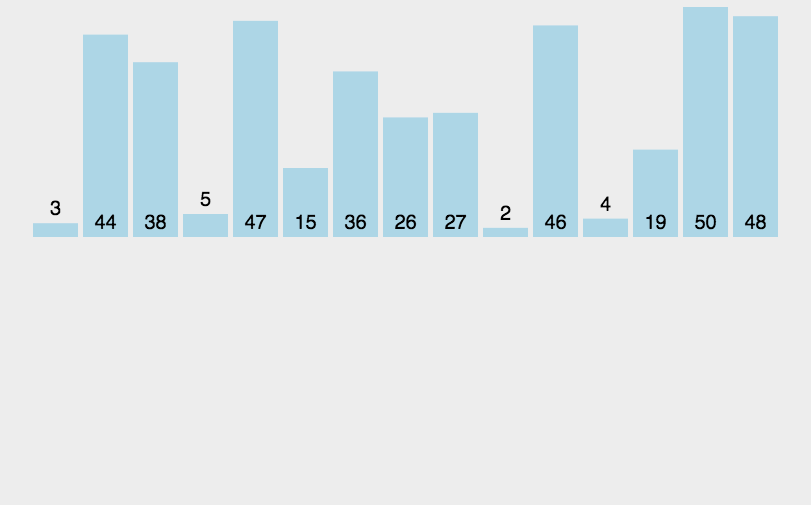``````public class InsertSort {
public static void insertSort(int array[]) {
if (array == null || array.length == 0) {
return;
}
for (int i = 1; i < array.length; i++) {
// 当array[i]比arra[i - 1]小时才进入处理
if (array[i] < array[i - 1]) {
// 临时变量存储array[i]的值
int temp = array[i];
// 从当前位置开始处理
int j = i;
// 将比temp大的数往后挪一个位置，为temp腾出一个合适位置
while (j > 0 && temp < array[j - 1]) {
array[j] = array[j - 1];
j--; // 填充完后, 继续向前比较
}
// 将temp放在属于它的位置上
array[j] = temp;
}
}
}
}``````

1. 在最坏的情况下，即整个数组是倒序的，比较次数 = 1 + 2 + 3 + ... + (n - 2) + (n - 1) = n * (n - 1) / 2，此时的时间复杂度为：O(n^2)。
2. 在最好的情况下，即整个数组是正序的，比较次数 = n - 1，此时的时间复杂度为：O(n)。
• 选择排序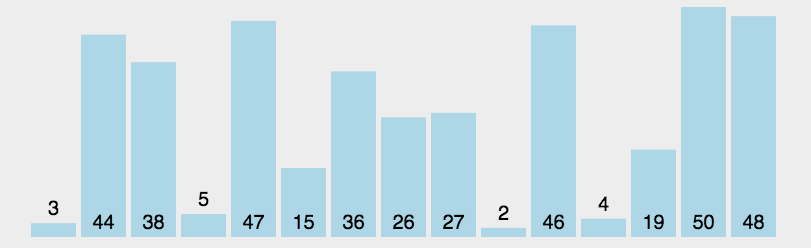``````public class SelectSort {
public static void selectSort(int[] array) {
if (array == null || array.length == 0) {
return;
}
for (int i = 0; i < array.length - 1; i++) {
// 记录当次遍历值最小的数的位置
int minIndex = i;
for (int j = i + 1; j < array.length; j++) {
// 如果array[j]比array[minIndex]小, 则将minIndex修改为j
if (array[j] < array[minIndex]) {
minIndex = j;
}
}
if (i != minIndex) {
// 将当次遍历的最小值交换到对应位置
int temp = array[i];
array[i] = array[minIndex];
array[minIndex] = temp;
}
}
}
}``````

• 快速排序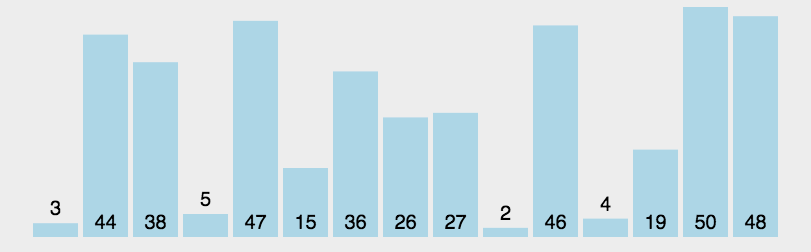``````public class QuickSort {
private static void quickSort(int[] array, int low, int high) {
if (low >= high) {
return;
}
int i = low, j = high, index = array[i]; // 取最左边的数作为基准数
while (i < j) {
while (i < j && array[j] >= index) { // 向左寻找第一个小于index的数
j--;
}
if (i < j) {
array[i++] = array[j]; // 将array[j]填入array[i]，并将i向右移动
}
while (i < j && array[i] < index) {// 向右寻找第一个大于index的数
i++;
}
if (i < j) {
array[j--] = array[i]; // 将array[i]填入array[j]，并将j向左移动
}
}
array[i] = index; // 将基准数填入最后的坑
quickSort(array, low, i - 1); // 递归调用，分治
quickSort(array, i + 1, high); // 递归调用，分治
}

public static void quickSort(int[] array) {
if (array == null || array.length == 0) {
return;
}
quickSort(array, 0, array.length - 1);
}
}``````

1. 最好情况的时间复杂度为O(nlogn)。
2. 最差情况下每一次取到的数（基准数）都是当前要比较的数中的最大/最小值，在这种情况下，每次都只能得到比上一次少1个数的子序列（即要么全比基准数大，要么全比基准小），此时相当于一个冒泡排序，比较的次数 = (n - 1) + (n - 2) + ... + 2 + 1 = (n - 1) * n / 2，此时的时间复杂度为：O(n^2)。最差情况一般出现在：待排序的数据本身已经是正序或反序排好了。
• 归并排序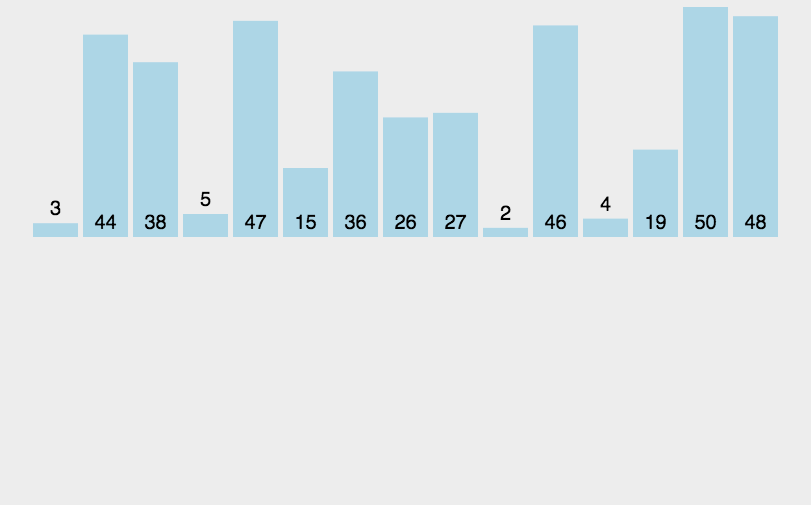``````public class MergeSort {
public static void mergeSort(int[] array) {
if (array == null || array.length == 0)
return;
int[] temp = new int[array.length];
mergeSort(array, 0, array.length - 1, temp);

}
// 归并
private static void mergeSort(int array[], int first, int last, int temp[]) {
if (first < last) {
int mid = (first + last) / 2;
mergeSort(array, first, mid, temp); // 递归归并左边元素
mergeSort(array, mid + 1, last, temp); // 递归归并右边元素
mergeArray(array, first, mid, last, temp); // 再将二个有序数列合并
}
}

/**
* 合并两个有序数列
* array[first]~array[mid]为第一组
* array[mid+1]~array[last]为第二组
* temp[]为存放两组比较结果的临时数组
*/
private static void mergeArray(int array[], int first, int mid, int last, int temp[]) {
int i = first, j = mid + 1; // i为第一组的起点, j为第二组的起点
int m = mid, n = last; // m为第一组的终点, n为第二组的终点
int k = 0; // k用于指向temp数组当前放到哪个位置
while (i <= m && j <= n) { // 将两个有序序列循环比较, 填入数组temp
if (array[i] <= array[j])
temp[k++] = array[i++];
else
temp[k++] = array[j++];
}
while (i <= m) { // 如果比较完毕, 第一组还有数剩下, 则全部填入temp
temp[k++] = array[i++];
}
while (j <= n) {// 如果比较完毕, 第二组还有数剩下, 则全部填入temp
temp[k++] = array[j++];
}
for (i = 0; i < k; i++) {// 将排好序的数填回到array数组的对应位置
array[first + i] = temp[i];
}
}
}``````